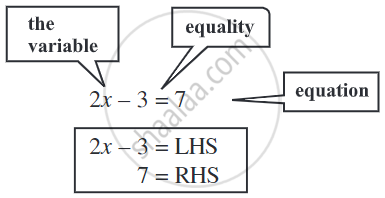# Linear Equation in One Variable

#### definition

• Linear Equation in One Variable: If there is only one variable in the equation then it is called a linear equation in one variable.

# Linear Equation in One Variable:

If there is only one variable in the equation then it is called a linear equation in one variable. These are called linear equations in one variable because the highest degree of the variable is one.

These are linear expressions:

2x, 2x + 1, 3y – 7, 12 – 5z, 5/4(x - 4) + 10

These are not linear expressions:
x2 + 1, y + y2, 1 + z + z2 + z3.     ....(since highest power of variable > 1)

The general form is ax + b = c, where a, b and c are real numbers and a ≠ 0.

Example:

x + 5 = 10, y – 3 = 19.

### Some Important points related to Linear Equations:• There is an equality sign in the linear equation. The expression on the left of the equal sign is called the LHS (left-hand side) and the expression on the right of the equal sign is called the RHS (right-hand side).

• In the linear equation, the LHS is equal to RHS but this happens for some values only and these values are the solution of these linear equations.

If you would like to contribute notes or other learning material, please submit them using the button below.

### Shaalaa.com

Linear Equations & Linear Equations in One Variable [00:22:54]
S
0%